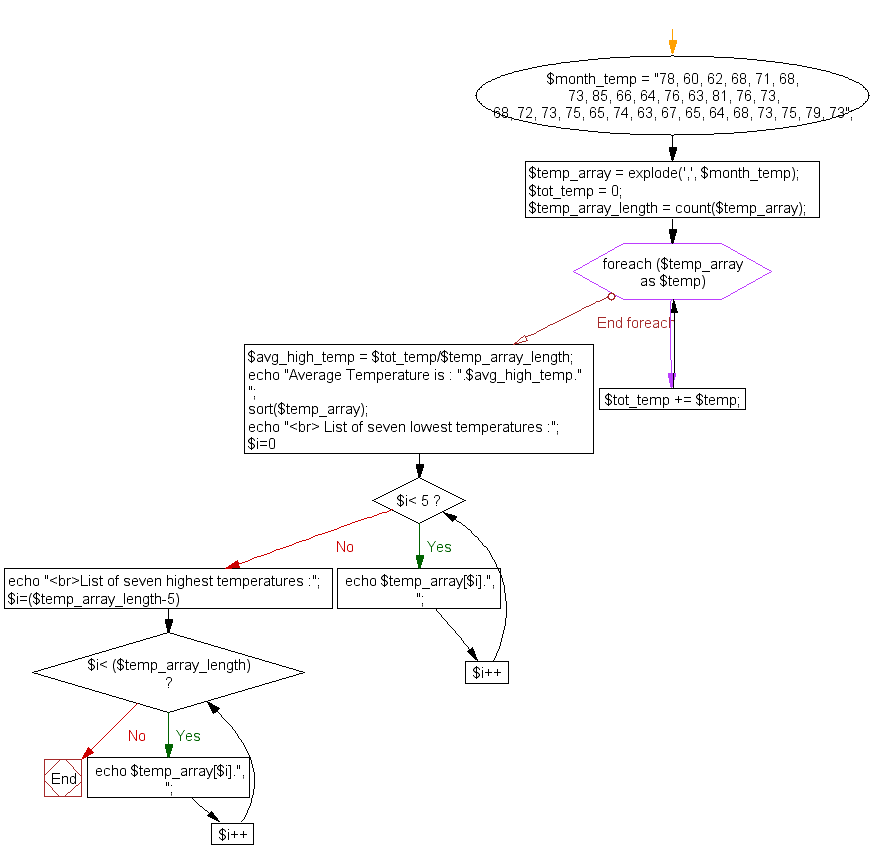﻿ PHP Array Exercise: Calculate and display average temperature - w3resource# PHP Array Exercises : Calculate and display average temperature

## PHP Array: Exercise-9 with Solution

Write a PHP script to calculate and display average temperature, five lowest and highest temperatures.

Recorded temperatures : 78, 60, 62, 68, 71, 68, 73, 85, 66, 64, 76, 63, 75, 76, 73, 68, 62, 73, 72, 65, 74, 62, 62, 65, 64, 68, 73, 75, 79, 73

Sample Solution:

PHP Code:

``````<?php
\$month_temp = "78, 60, 62, 68, 71, 68, 73, 85, 66, 64, 76, 63, 81, 76, 73,
68, 72, 73, 75, 65, 74, 63, 67, 65, 64, 68, 73, 75, 79, 73";
\$temp_array = explode(',', \$month_temp);
\$tot_temp = 0;
\$temp_array_length = count(\$temp_array);
foreach(\$temp_array as \$temp)
{
\$tot_temp += \$temp;
}
\$avg_high_temp = \$tot_temp/\$temp_array_length;
echo "Average Temperature is : ".\$avg_high_temp."
";
sort(\$temp_array);
echo " List of five lowest temperatures :";
for (\$i=0; \$i< 5; \$i++)
{
echo \$temp_array[\$i].", ";
}
echo "List of five highest temperatures :";
for (\$i=(\$temp_array_length-5); \$i< (\$temp_array_length); \$i++)
{
echo \$temp_array[\$i].", ";
}
?>
```
```

Sample Output:

```Average Temperature is : 70.6
List of five lowest temperatures : 60,  62,  63,  63,  64,
List of five highest temperatures : 76, 78,  79,  81,  85,
```

Flowchart:PHP Code Editor:

What is the difficulty level of this exercise?

Test your Programming skills with w3resource's quiz.

﻿

## PHP: Tips of the Day

In PHP, there are two versions of logical AND and OR operators.

Operator True if
\$a and \$b Both \$a and \$b are true
\$a && \$b Both \$a and \$b are true
\$a or \$b Either \$a or \$b is true
\$a || \$b Either \$a or \$b is true

Note that the && and || opererators have higher precedence than and and or. See table below:

Evaluation Result of \$e Evaluated as
\$e = false || true True \$e = (false || true)
\$e = false or true False (\$e = false) or true

Because of this it's safer to use && and || instead of and and or.